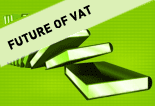Information collected by Member States on a national basis National Procedure codes(D.E. 1/11-Box 37) National codes for certificates, documents and authorizations(D.E. 2/3- Box 44) National codes for additional information(D.E. 2/2-Box 44) National codes for type of tax(D.E. 4/3-Box 47) BEBGEN BG+EN BG+EN EN BG+EN CZEN EN EN+CZ EN EN +CZ DKDA DA DA DEDE DE DE EN DE EEEN EE EE+EN EN IEEN EN EN EN EN ELESESEN FRFR FR FR FR+EN ITIT IT IT IT IT CYEN EN +EL EN EN +EL EN LVLV LV LV LV LTEN LT EN LUHUEN EN+HU EN+HU EN+HU HU HRHR+EN HR+EN HR+EN HR MTEN EN EN EN NLNL+EN NL NL NL NL ATEN DE+EN DE+EN DE+EN DE+EN PLEN EN EN EN EN PTEN PT EN EN PT RORO RO EN RO SISL EN+SL EN+SL EN SKEN SK+EN SK SK+EN SK+EN FIEN EN+FI EN+FI EN+FI EN+FI SESV+EN SV+EN SV+EN UKEN EN EN EN